# Unique factorization domain that is not a field has either infinitely many units or infinitely many associate classes of irreducibles

## Statement

Suppose$R$ is a unique factorization domain that is not a field. Then, at least one of these is true for$R$:

•$R$ has infinitely many units.
•$R$ has infinitely many associate classes of irreducible elements.

## Related facts

### Particular cases

• In the ring of rational integers, there are only finitely many units, and thus, there are infinitely many associate classes of primes. This is the well-known fact that there are infinitely many primes.
• In the formal power series ring over a field, there is only one associate class of irreducibles, but there are infinitely many units.

### Generalizations

The hypothesis can be weakened slightly: it works for any integral domain that is also a ring satisfying ACCP: this is precisely the condition needed to ensure that every element does have a factorization, not necessarily unique. Further information: Integral domain satisfying ACCP that is not a field has either infinitely many units or infinitely many associate classes of irreducibles

## Proof

Given: A unique factorization domain$R$ that is not a field.

To prove:$R$ either has infinitely many units or infinitely many irreducible elements.

Proof: For the proof, we assume that$R$ has only finitely many units, and prove that$R$ has infinitely many irreducible elements.

Since$R$ is not a field, it has at least one irreducible element, say$p_1$. Suppose now that the set of associate classes of irreducible elements is finite, say with representatives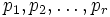$p_1,p_2, \dots, p_r$ for the associate classes. Let$a$ be the product of the$p_i$s. Consider now the set:$S = \{ 1 + a, 1 + a^2, 1 + a^3, \dots \}$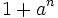$1 + a^n$ cannot be equal to zero for any$n$, because that would imply that$a$ is a unit, forcing$p_i$ to be units, a contradiction to irreducibility. For a similar reason,$a^n$ cannot ever be$1$.

Since the$p_i$ are not zero,$a$ is not zero, and we have$(1 + a^m) - (1 + a^n) = a^m(1 - a^{n-m})$ which is nonzero since both$a$ and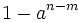$1 - a^{n-m}$ are nonzero, and$R$ is an integral domain. Thus, all the$1 + a^n$ are distinct. Thus,$S$ is an infinite set of distinct nonzero elements.

Since there are only finitely many units in$R$, there exists$n$ such that$1 + a^n$ is not a unit. Since$R$ is a unique factorization domain,$1 + a^n$ has a factorization into irreducibles, and in particular, has at least one irreducible factor. However, if$p_i$ divides$1 + a^n$ for any$i$, we have that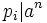$p_i | a^n$ and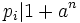$p_i | 1 + a^n$, so$p_i | 1$, a contradiction. Thus, the assumption that there are only finitely many associate classes of irreducible elements is flawed.